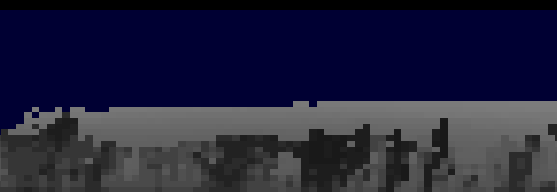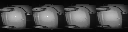# Creating a grayscale png image from a vector of int.

• Hello All,
I have an vector of integers(approx count of 4096) of which values range from 0 - 4000.
I want to create a grayscale png image(128*32 resolution = 4096). I know that grayscale values range from 0-255

1. Is there a direct way to convert a vector to PNG image.
2. My values range from 0-4000. How can these values be converted to grayscale i.e 0-255
3. Below is the image I need.• @Uday-More

Is there a direct way to convert a vector to PNG image.

yes, if the (Q?)vector has the correct format. Your's does not and so you'll need to do some modifications on your data first.

My values range from 0-4000. How can these values be converted to grayscale i.e 0-255

You'll have to map your data accordingly

Example:

``````int main(int argc, char *argv[])
{
QApplication a(argc, argv);

QVector<int>numbers( 4096, 2000); //vector of size 4096 filled (in this example) with all 2000

auto mapRange = [](int input)->quint8 {
//Mapping follows: Y = (X-minRangeOld)/(maxRangeOld-minRangeOld) * (maxRangeNew-minRangeNew) + minRangeNew
return static_cast<quint8>(input /4000.0 * 255);
};

QVector<quint8> mappedData(4096);
for(int i(0); i < 4096; i++){
mappedData[i] = mapRange(numbers.at(i));
}

QImage greyScaleImage(mappedData.data(), 128, 32, 128, QImage::Format_Grayscale8);

QLabel l;
l.resize(128,32);
l.setPixmap(QPixmap::fromImage(greyScaleImage));
l.show();

return a.exec();
}
``````

• return static_cast<quint8>(input /4000.0 * 255);

This should be return static_cast<quint8>(input /4096.0 * 255);

Able to generate images now.

• This should be ... 4096.0 ....

why ? you wrote:

values range from 0 - 4000.

Able to generate images now.

Great ! Don't forget to use the topic tools to set the topic to solved then

• @J-Hilk . Thnx for the input.
I have a doubt arranging the vector of the "mappedData"
We are forming the image with width 128 and height as 32 and the 3rd argument is "bytesPerLine" which was given as 128.
What is the importance of "bytesperline".

Generated Images are below.It is repeating the sections of image.

• @Uday-More

What is the importance of "bytesperline".

well its part of the constructor :P

for a Format_Grayscale8 Image, the bytes per line is equal to your pixels for your width,
for a Format_Grayscale16 image, the bytes per line would be double the pixels for your width, because your Pixel information is composed of 2 bytes instead of 1

• @J-Hilk . I tried to convert the values from 0-65536 (16 bit)

1. There is no Format_Grayscale16 . It is written (// reserved for future use). Therefore, I use Format_RGB16. Is it correct.
2. I used a QVector<quint16>. It gives be a build error "QImage::QImage(QImage &&) noexcept': cannot convert argument 1 from 'unsigned short *' to 'uchar *".

What type of vector should be used in such cases to have values ranging from 0-65536 ?

• Format_RGB16

You'll need to know exactly, what you want to do and what you're doing.

Format_RGB16 means, It expects a 16 bit value for Red and 16 bit value for Green and 16 bit value for Blue -> 46 bits (6Bytes) per pixel -> 768 BytesperLine (with a width of 128 from before)

Therefore your seeder QVector<quint16> needs to have a size of 12.288. Does it?

I used a QVector<quint16>. It gives be a build error "QImage::QImage(QImage &&) noexcept': cannot convert argument 1 from 'unsigned short *' to 'uchar *".

Please show the correlating code segment

What type of vector should be used in such cases to have values ranging from 0-65536?

I assume you mean 65535, because 65536 requires more than 16 bit.
QVector<quint16> sounds appropriate

1. I, earlier need 0-255 (8 bit) which worked properly
2. Yes Format_RGB16 is what I need now. Similar to image given below. We can see there is blue element.1. I meant 65535

4)As explained earlier, i derived this statement of
QVector<quint16> mappedData;
QImage greyScaleImage(mappedData.data(), 32, 128, 768, QImage::Format_RGB16);

This gives me an error "QImage::QImage(QImage &&) noexcept': cannot convert argument 1 from 'unsigned short *' to 'uchar *"

From qglobal.h header i found this.
typedef unsigned char quint8; /* 8 bit unsigned /
typedef unsigned short quint16; /
16 bit unsigned */

• greyScaleImage

This is not clear to me: if this is a greyscale image how can it have blue color?
What format is it actually: 16bit per pixel greyscale? Or RGB with 16bit per color channel?

• 768

Code goes below

``````// Fetching the intensity values from the cloud
int pointsSize = m_pCurrentSetCloud.get()->points.size();
QVector<float> intensityVector;
for(int i = 0; i < pointsSize; ++i)
{
intensityVector.push_back((m_pCurrentSetCloud.get()->points[i].intensity));
}

float maxValue = *std::max_element(intensityVector.begin(), intensityVector.end());

// Scaling the values to 0-65535
auto mapRange = [maxValue](float input)->quint16 {
return static_cast<quint16>((input / maxValue) * 65536);
};

QVector<quint16> mappedData(pointsSize);
for(int i(0); i < pointsSize; i++){
mappedData[i] = mapRange(intensityVector.at(i));
}

// Creating the 16bit RGB image and rotating it by 270 degrees fro display
QImage rgbImage(mappedData.data(), 32, 128, 768, QImage::Format_RGB16);
QImage rotatedImage = rgbImage.transformed(QMatrix().rotate(270.0));
rotatedImage.save("intensity.png");``````

• May be I am complicating a little bit. I don't have much knowledge on the QImage and bytes needed for information.

1. I generated a grayscale image previously from which i understand that each pixel is holding a 8 bit value (0-255). Similar to this can I have 8 bit RGB ? How will this be arranged ? How many bytes foreach component ? If we arrange this in 32*128, will it be 32 bytes per line ?

• If we arrange this in 32*128, will it be 32 bytes per line ?

No. It will be 32 * number_of_columns bytes.
I assume you mean QImage::Format_RGB32 which uses 32 bytes per pixel.

From the documentation: "QImage::Format_RGB32 4 The image is stored using a 32-bit RGB format (0xffRRGGBB)."
So, first byte 0xFF, then red, green, blue.